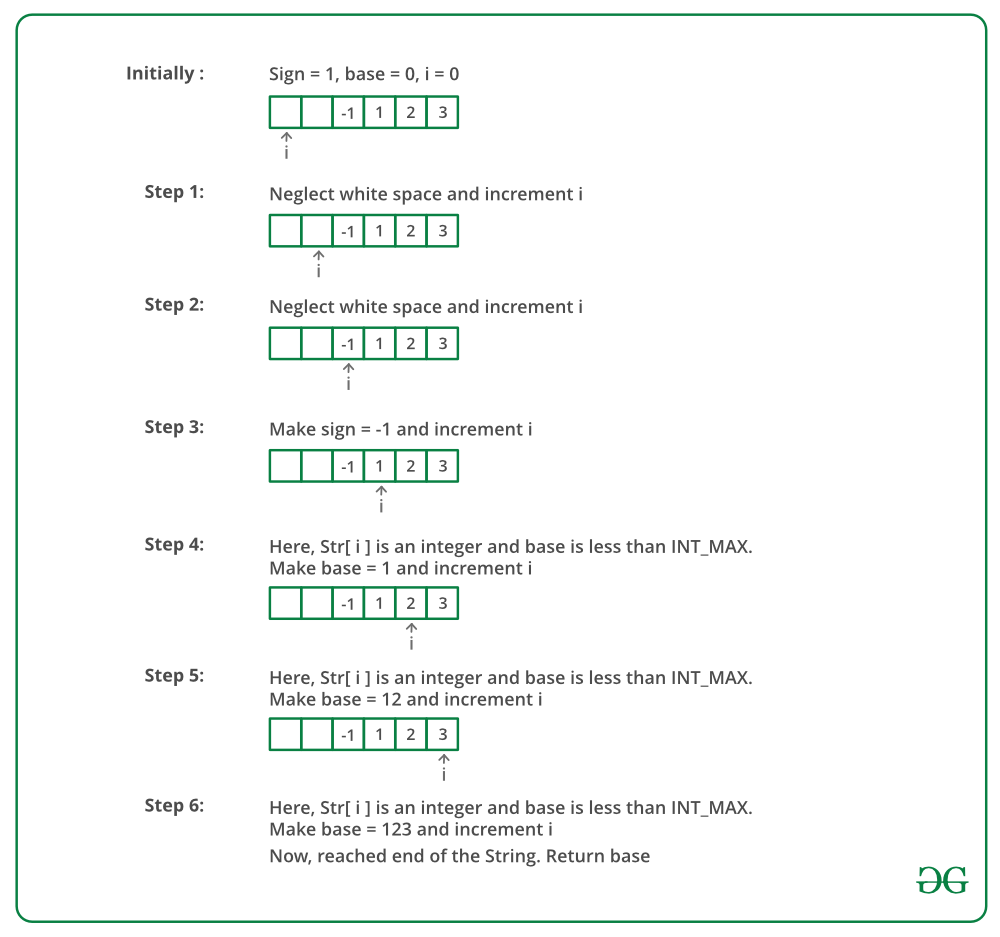GeeksforGeeks App
Open AppBrowser
Continue

# C++ Program To Write Your Own atoi()

The atoi() function in C takes a string (which represents an integer) as an argument and returns its value of type int. So basically the function is used to convert a string argument to an integer.

Syntax:

`int atoi(const char strn)`

Parameters: The function accepts one parameter strn which refers to the string argument that is needed to be converted into its integer equivalent.

Return Value: If strn is a valid input, then the function returns the equivalent integer number for the passed string number. If no valid conversion takes place, then the function returns zero.

Example:

## C++

 `#include ``using` `namespace` `std;` `int` `main()``{``    ``int` `val;``    ``char` `strn1[] = ``"12546"``;` `    ``val = ``atoi``(strn1);``    ``cout <<``"String value = "` `<< strn1 << endl;``    ``cout <<``"Integer value = "` `<< val << endl;` `    ``char` `strn2[] = ``"GeeksforGeeks"``;``    ``val = ``atoi``(strn2);``    ``cout <<``"String value = "` `<< strn2 << endl;``    ``cout <<``"Integer value = "` `<< val <

Output

```String value = 12546
Integer value = 12546
String value = GeeksforGeeks
Integer value = 0```

Time Complexity : O(N)

Auxiliary Space : O(1) , as no extra space is needed.

Now let’s understand various ways in which one can create their own atoi() function supported by various conditions:

Approach 1: Following is a simple implementation of conversion without considering any special case.

• Initialize the result as 0.
• Start from the first character and update result for every character.
• For every character update the answer as result = result * 10 + (s[i] – ‘0’)

## C++

 `// A simple C++ program for``// implementation of atoi``#include ``using` `namespace` `std;` `// A simple atoi() function``int` `myAtoi(``char``* str)``{``    ``// Initialize result``    ``int` `res = 0;` `    ``// Iterate through all characters``    ``// of input string and update result``    ``// take ASCII character of corresponding digit and``    ``// subtract the code from '0' to get numerical``    ``// value and multiply res by 10 to shuffle``    ``// digits left to update running total``    ``for` `(``int` `i = 0; str[i] != ``''``; ++i)``        ``res = res * 10 + str[i] - ``'0'``;` `    ``// return result.``    ``return` `res;``}` `// Driver code``int` `main()``{``    ``char` `str[] = ``"89789"``;``  ` `    ``// Function call``    ``int` `val = myAtoi(str);``    ``cout << val;``    ``return` `0;``}` `// This is code is contributed by rathbhupendra`

Output

`89789`

Approach 2: This implementation handles the negative numbers. If the first character is ‘-‘ then store the sign as negative and then convert the rest of the string to number using the previous approach while multiplying sign with it.

## C++

 `// A C++ program for``// implementation of atoi``#include ``using` `namespace` `std;` `// A simple atoi() function``int` `myAtoi(``char``* str)``{``    ``// Initialize result``    ``int` `res = 0;` `    ``// Initialize sign as positive``    ``int` `sign = 1;` `    ``// Initialize index of first digit``    ``int` `i = 0;` `    ``// If number is negative,``    ``// then update sign``    ``if` `(str == ``'-'``) {``        ``sign = -1;` `        ``// Also update index of first digit``        ``i++;``    ``}` `    ``// Iterate through all digits``    ``// and update the result``    ``for` `(; str[i] != ``''``; i++)``        ``res = res * 10 + str[i] - ``'0'``;` `    ``// Return result with sign``    ``return` `sign * res;``}` `// Driver code``int` `main()``{``    ``char` `str[] = ``"-123"``;` `    ``// Function call``    ``int` `val = myAtoi(str);``    ``cout << val;``    ``return` `0;``}` `// This is code is contributed by rathbhupendra`

Output

`-123`

Approach 3: This implementation handles various type of errors. If str is NULL or str contains non-numeric characters then return 0 as the number is not valid.

Output

` -134`

Approach 4: Four corner cases needs to be handled:

• Sign of the number
• Overflow
• Invalid input

To remove the leading whitespaces run a loop until a character of the digit is reached. If the number is greater than or equal to INT_MAX/10. Then return INT_MAX if the sign is positive and return INT_MIN if the sign is negative. The other cases are handled in previous approaches.

Dry Run:Below is the implementation of the above approach:

## C++

 `// A simple C++ program for``// implementation of atoi``#include ``using` `namespace` `std;` `int` `myAtoi(``const` `char``* str)``{``    ``int` `sign = 1, base = 0, i = 0;``    ` `    ``// if whitespaces then ignore.``    ``while` `(str[i] == ``' '``)``    ``{``        ``i++;``    ``}``    ` `    ``// sign of number``    ``if` `(str[i] == ``'-'` `|| str[i] == ``'+'``)``    ``{``        ``sign = 1 - 2 * (str[i++] == ``'-'``);``    ``}``  ` `    ``// checking for valid input``    ``while` `(str[i] >= ``'0'` `&& str[i] <= ``'9'``)``    ``{``        ``// handling overflow test case``        ``if` `(base > INT_MAX / 10``            ``|| (base == INT_MAX / 10``            ``&& str[i] - ``'0'` `> 7))``        ``{``            ``if` `(sign == 1)``                ``return` `INT_MAX;``            ``else``                ``return` `INT_MIN;``        ``}``        ``base = 10 * base + (str[i++] - ``'0'``);``    ``}``    ``return` `base * sign;``}`  `// Driver Code``int` `main()``{``    ``char` `str[] = ``"  -123"``;``  ` `    ``// Functional Code``    ``int` `val = myAtoi(str);``    ``cout <<``" "``<< val;``    ``return` `0;``}` `// This code is contributed by shivanisinghss2110`

Output

` -123`

Complexity Analysis for all the above Approaches:

• Time Complexity: O(n).
Only one traversal of string is needed.
• Space Complexity: O(1).
As no extra space is required.

Exercise:
Write your won atof() that takes a string (which represents an floating point value) as an argument and returns its value as double.

Please refer complete article on Write your own atoi() for more details!

My Personal Notes arrow_drop_up